# 你看我还有机会吗？【Python基础速览1】变量 | 输出和输入 | 数字类型 | 字符串类型

1年前   阅读数 111 0## 前言：## 一、变量

### 0x00 变量的定义

📚 Python 在定义变量时，并不需要声明变量类型。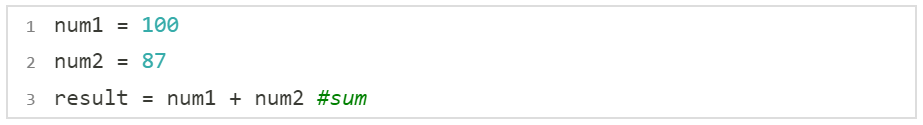### 0x01 变量的类型

📚 为了更充分地利用内存空间，更有效率地管理内存，变量有不同的类型。Python中的对象分类：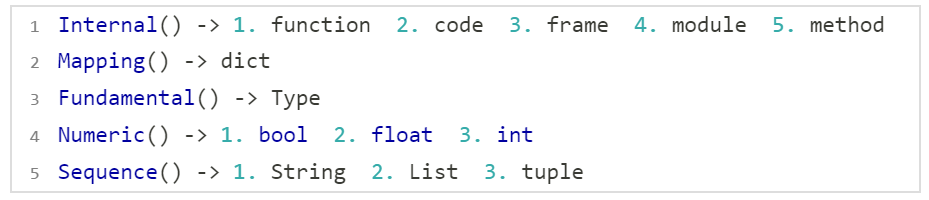### 0x02 查看变量类型

📚 type() 获取变量类型：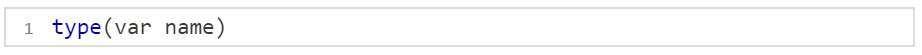💬 代码演示：

``````a = 10;
print(a, type(a))``````

🚩 运行结果： 10  <class 'int'>

### 0x03 赋值方式

📚 一般赋值：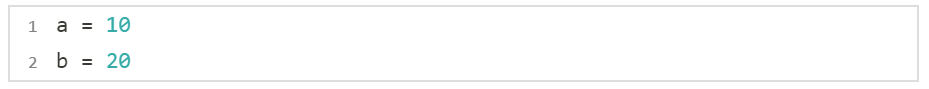📚 链式赋值：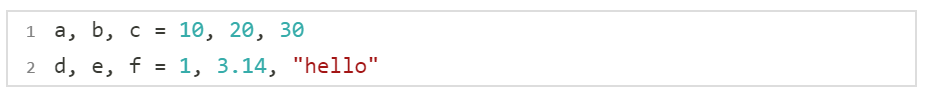💬 交换两个变量：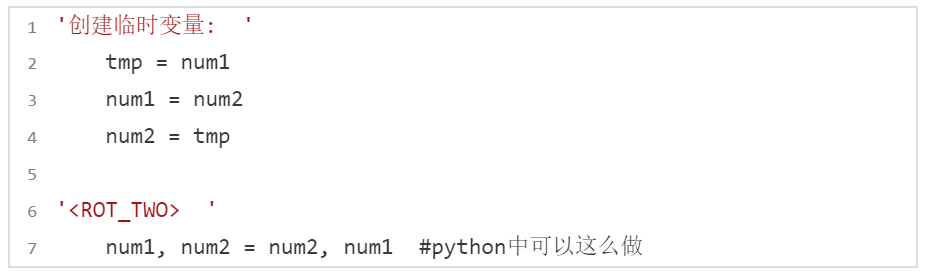## 二、标识符和关键字

### 0x00 标识符的命名规则

📚 Python 中的标识符是区分大小写的！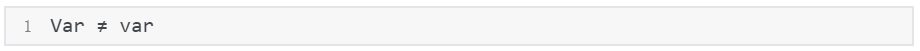### 0x01 命名规则和方法

📚 大驼峰命名法、小驼峰命名法……  Python 中推荐使用下划线 "_"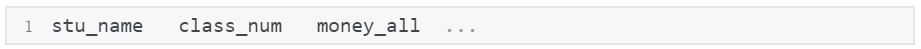### 0x02 关键字（保留字）

📚 查看关键字：（这里 import 可以先理解为C语言中的 #include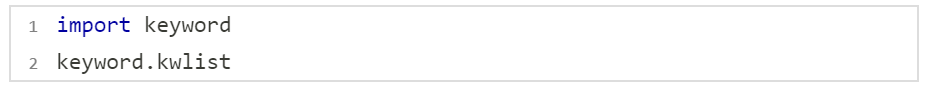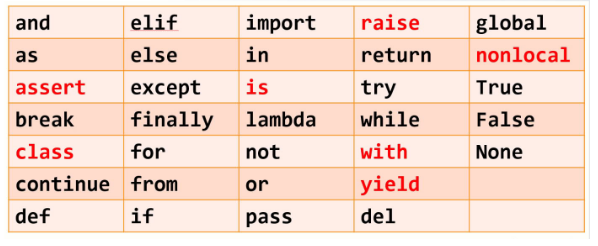## 三、Python的一些性质

### 0x00 垃圾回收机制

``````name = user = "hello"
👆 变量name，变量user指向常量“hello”这个内存``````

📚 查看 id 的方式：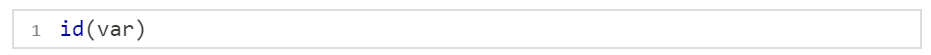将 id(name) 和 id(user) 对比后可以发现，他们指向的 id 相同，那么说明有两个指针指向 "hello" 这个内存！

📚 删除：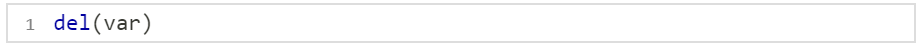💬 将 name 和 user 删除后我们再次访问 name 和 user，结果如下：

``````del(name)
del(user)``````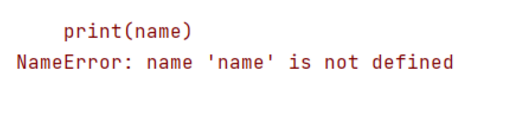💡 因为指向 "hello" 的所有指针已经被删除了，此时引用计数为0！

### 0x01 Python 弱类型语言

📚 Python 是一个弱变量的语言，Python的变量即用即生成，变量的类型由值来决定。

``````A = 1
A = "one"``````

## 四、输出和输入

### 0x00 print 输出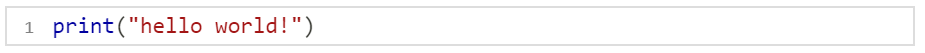### 0x01 input 输出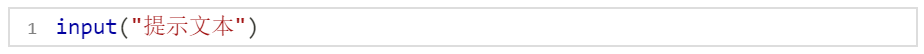### 0x03 Python3 版本

raw_input() 被摒弃，保留了 input()，python3 中的 input 继承了 raw_input 的效果

💬 在Python3中，不 print 就不会打印：

``````name = input("請輸入姓名：")
print(name)``````

### 0x04 input "切分"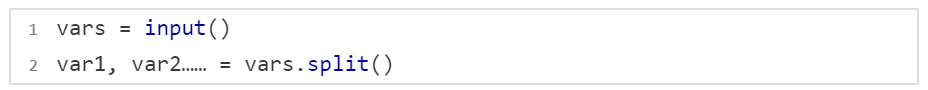``````names = input("请输入两个名字: ")
n1, n2 = names.split()
print("第一个名字:" + n1, "第二个名字:" + n2)``````

⚡ 还可以这么写：

``n1, n2 = input("请输入两个名字：").split()``

## 四、Python数字类型

### 0x00 数字类型介绍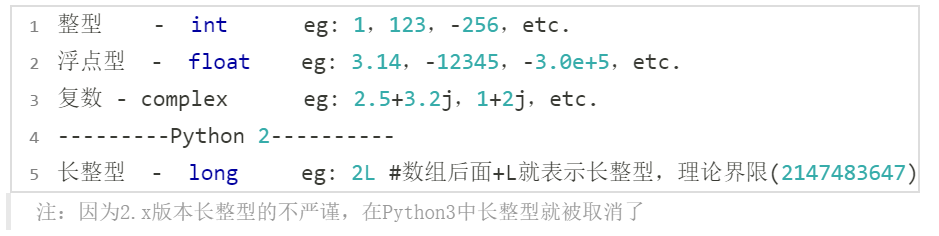📚 关于复数：

``````x = 2+3j
y = 7j
z = -7j

print(type(x))
print(type(y))
print(type(z))``````

### 0x01 数据类型转换

📚 查看数据类型：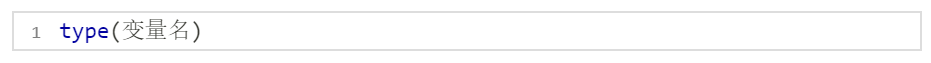💬 代码演示：

``````print(type(1))
print(type(1.0))``````

🚩  <class 'int'>

<class 'float'>

### 0x02 数字类型运算符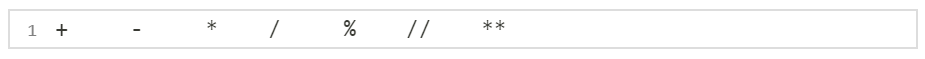📚 // 取整数 （抛弃余数）：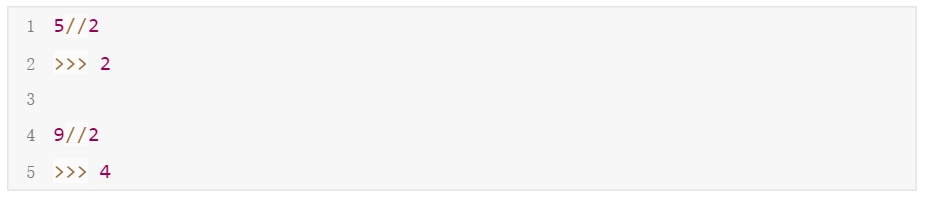📚 ** 幂（符号^ ，次方）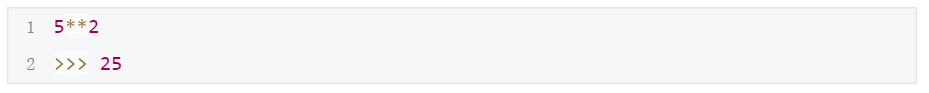### 0x03 复合运算符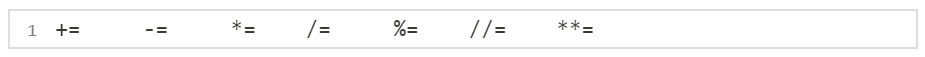###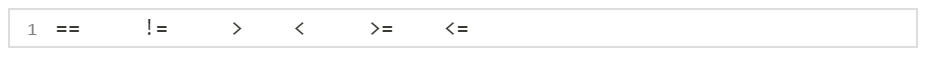💬 代码演示：

``````a, b = 10;
print(a==b)

print(a!=b)

print(a>b, a<b)

print(a<=b, a>=b)``````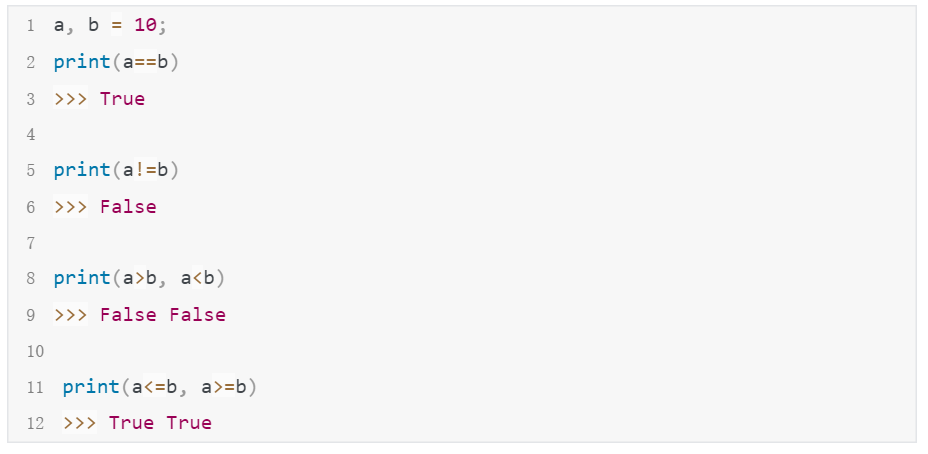### 0x05 逻辑运算符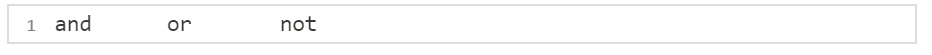📚 逻辑运算符的用法：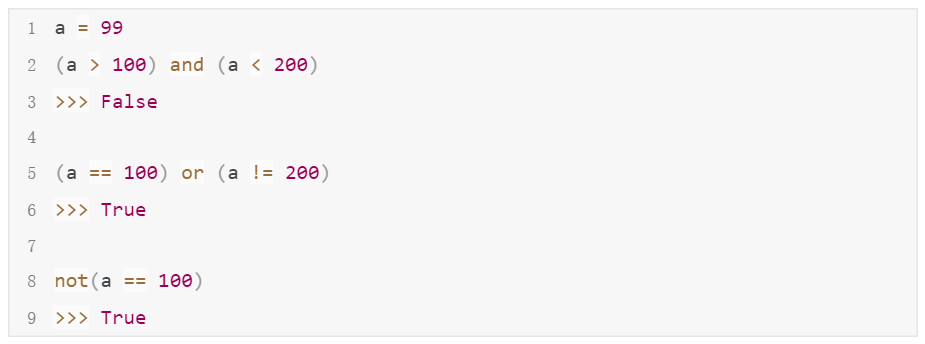💬 判断是否是闰年：

``````year = 2021
if ((year % 4 == 0) and (year % 100 != 0)) or (year % 400 == 0):
print("閏年")
else:
print("不是閏年")
``````

### 0x06 字符串运算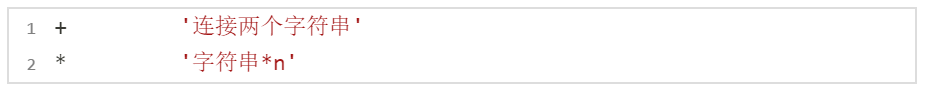💬 代码演示：

``````str1 = "hello" + "world"
print(str1)

str2 = "a" * 5
``````

🚩  helloworld

aaaaa

### 0x07 成员运算

📚 检测一个数据是否在指定的容器中：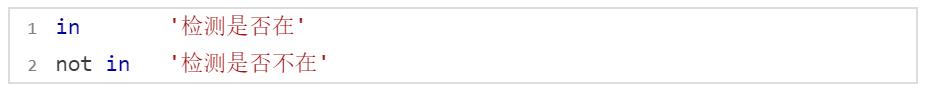💬 代码演示：

``````str1 = "hello world"
str2 = "hello"
print(str2 in str1)
# >>> True

str1 = "hello world"
str2 = 'z'
print(str2 not in str2)
# >>> True``````

🚩  True

True

### 0x08 优先级、

📚 优先级表（由高到低排序）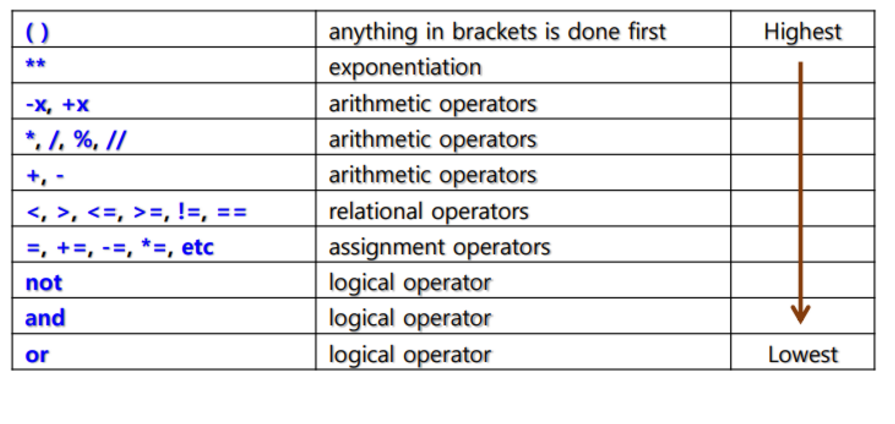### 0x09 Python内建函数（待补充）

📚 内建数值型函数：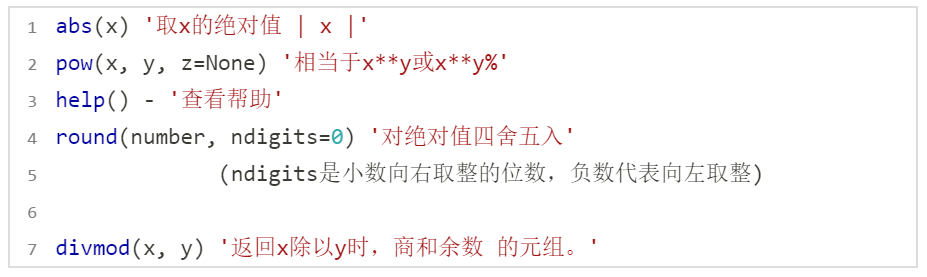💬 代码演示：

``````a = pow(2, 3) //#    2**3
b = pow(2, 3, 3) //#  2**3 % 3
print(a)
print(b)

#>>> 8
#    2

res = divmod(17, 4)
print(res)

#>>> (4, 1) #4……1``````

### 0x0A 强制类型转换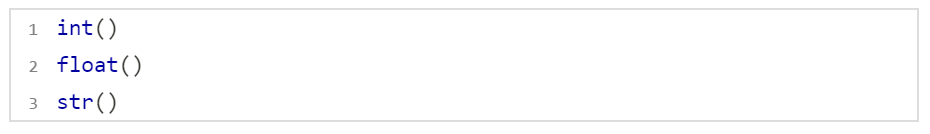## 五、字符串类型

### 0x00 Python 字符串

📚 在Python中，双引号或者单引号中的数据，就是字符串。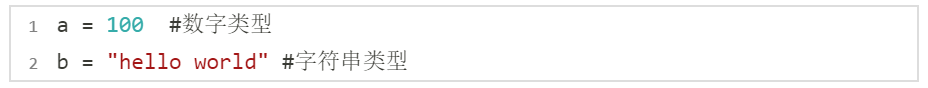### 0x01 引号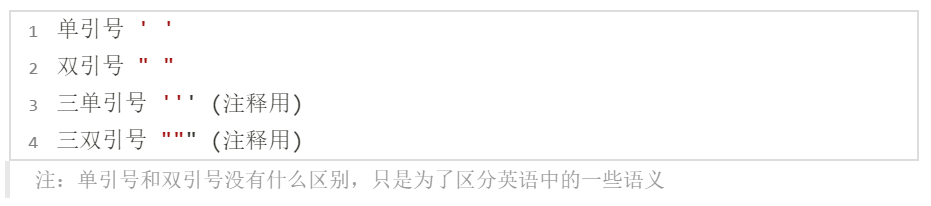💬 演示：

``````print("hello world")
'''
hello
nice to meet you
'''

"""
C, C++, Python
Java, Go
"""``````

### 0x02 字符串当中的特殊字符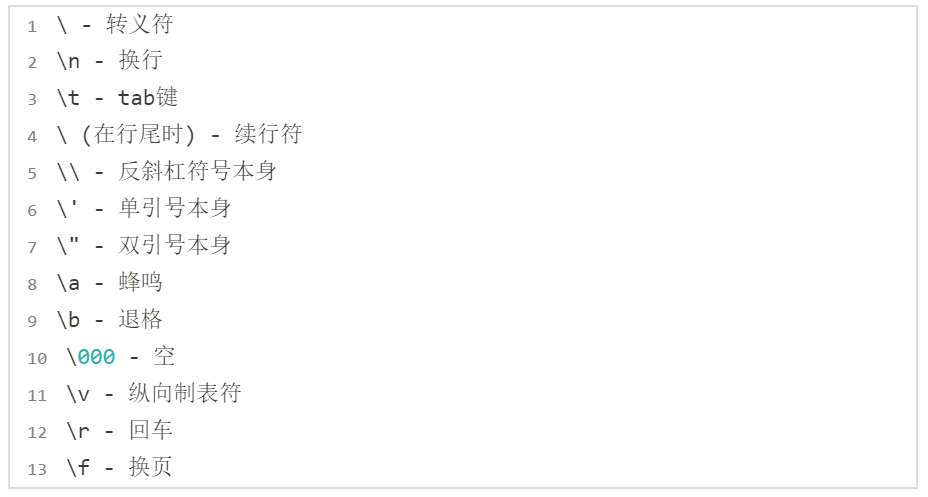### 0x03 字符串格式化操作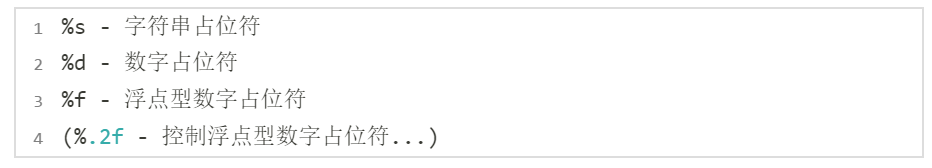💬 字符串格式化显示：

``````# 打印字符串&整数
print("my name is %s, age is %d" % ("xiaoming", 17))

# 打印浮点数
print("my height is %f" % (1.70))

# 变量形式
hobby = "sleep"
print("and my hobby is %s" % hobby)``````

### 0x04 索引 - index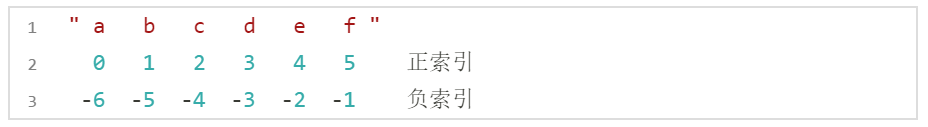📚 取单个元素 [ ]  ：

``print( "abcdef" )  #正取``

🚩  'c'

📚 字符串截取 [start:end] ：包头不包尾原则，为数学中的集合区间相同

``````a = "abcdef"
print( a[1:3] )
print( a[:] ) #截取所有字符串
print( a[:3]) #从开头截取到3前面为止
print( a[3:]) #从3截取到字符串的最后``````

🚩  'bc'

'abcdef'

'abc'

'def'

📚 步长截取 [start:end:step]：

``````str1 = "hello world"
print( str1[0:7:2] ) # [:7:2] '0可以省略'``````

🚩   'hlow'

📚 默认取法 string[start:end:step] 这三个参数都有默认值
start的默认值为0；end默认值为字符串结尾元素；step默认值为1；

``````str1 = "hello world"
print( str1[-1] )
print( str1[-3] )``````

🚩  'd'  'r'

📚 反字符串截取、步长截取：

``````str1 = "hello world"
print( str1[::-1] ) #反取整个字符串
print( str1[::-2] ) #反2步长截取
print( str1[1:9][::-1] )``````

🚩    'dlrow olleh'

'drwolh'

'row olle'

💬 演示：

``````str1 = "瞬美目以流眄 ，含言笑而不分"
print( str1[-3] + str1[-2] + str1[-1] ) #取字符串拼接
print( str1[-3:] ) # 利用负索引表示
print( str1[0:4] ) # 等同于str[:4] 0可省略
print( str1[:] ) # 全取
print( str1[4:0:-1] ) #倒取 4321（不包括0）
print( str1[::2] ) #跳2取
print( str1[::-1] ) #全部颠倒``````

🚩

我有话说: